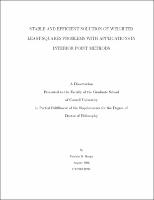## Stable and Efficient Solution of Weighted Least-Squares Problems with Applications in Interior Point Methods##### Abstract
In this thesis, we consider two closely related problems. The first is a full-rank weighted least-squares problem with a weight matrix that is positive definite, diagonal, and extremely ill conditioned. The ill-conditioning can cause standard algorithms to compute solutions with, in some cases, no digits of accuracy. Theory suggests the existence of an algorithm that will compute an accurate solution despite the ill-conditioning in the weight matrix. We describe a new algorithm, the Complete Orthogonal Decomposition (COD) Algorithm, for solving the weighted least-squares problem and show that it has this desirable property. In addition, the COD Algorithm is based on standard, well-understood techniques and is straightforward to implement. A natural application for the weighted least-squares problem described in the previous paragraph is interior point methods for linear programming. We discuss the problem in this context, and describe how the COD algorithm can be extended and used in this setting. Unlike other algorithms, this one is stable for interior point methods without assuming nondegeneracy in the linear programming instance. Computational experiments indicate that it is more reliable than other algorithms when the problem is near degenerate. The second problem involves a particular interior point algorithm. In 1994, Vavasis and Ye proposed a new primal-dual path-following interior point method, the layered-step interior point (LIP) method. This algorithm interleaves traditional steps with longer, layered least-squares (LLS) steps. Computation of a LLS step requires solving a weighted least-squares problem similar to the one described above, but the weight matrix also has the property that the weights fall into well-separated groups. This additional structure allows the problem to be broken down into smaller, constrained problems with well-conditioned weight matrices. The smaller problems can then be solved stably with standard algorithms, and the LLS step can be computed. Vavasis and Ye did not propose a particular algorithm for solving the LLS problem. In this thesis, we present an algorithm based on Cholesky factorization. The algorithm is such that a modified version of the sparse Cholesky code of Ng and Peyton of Oak Ridge National Laboratories can be used. Thus, the theoretical results are straight forward, and this algorithm proves to be accurate and efficient in practice.
1996-08
##### Publisher
Cornell University
theory center
##### Previously Published As
http://techreports.library.cornell.edu:8081/Dienst/UI/1.0/Display/cul.tc/96-256
technical report